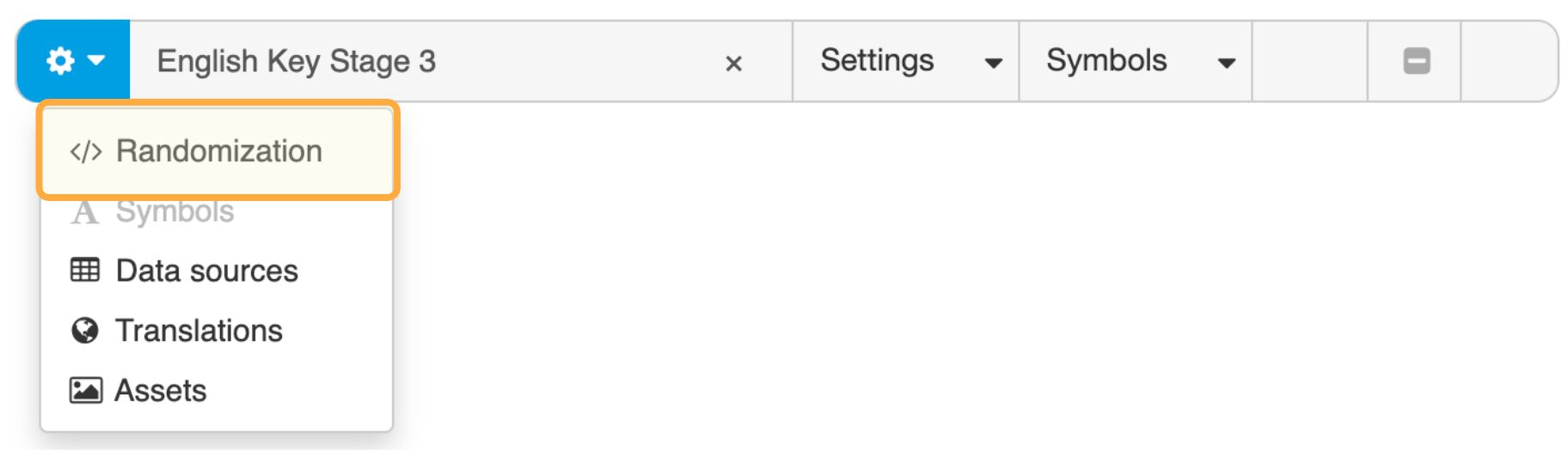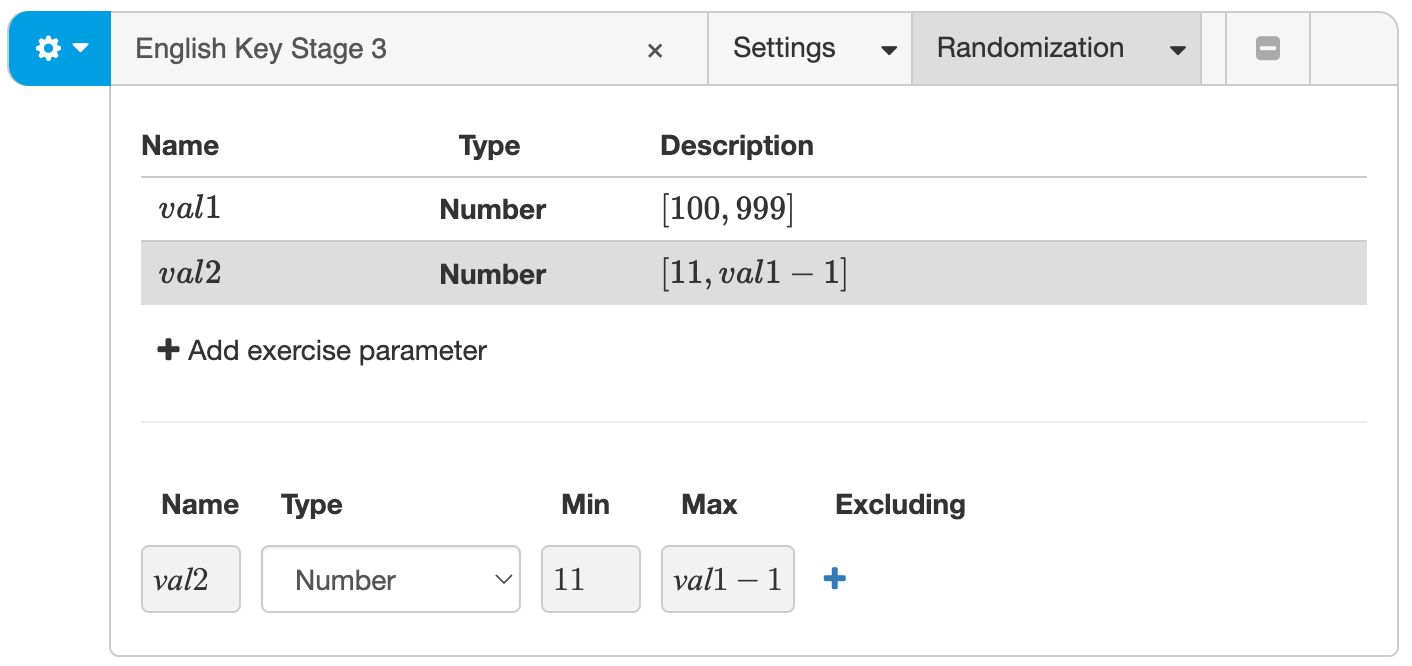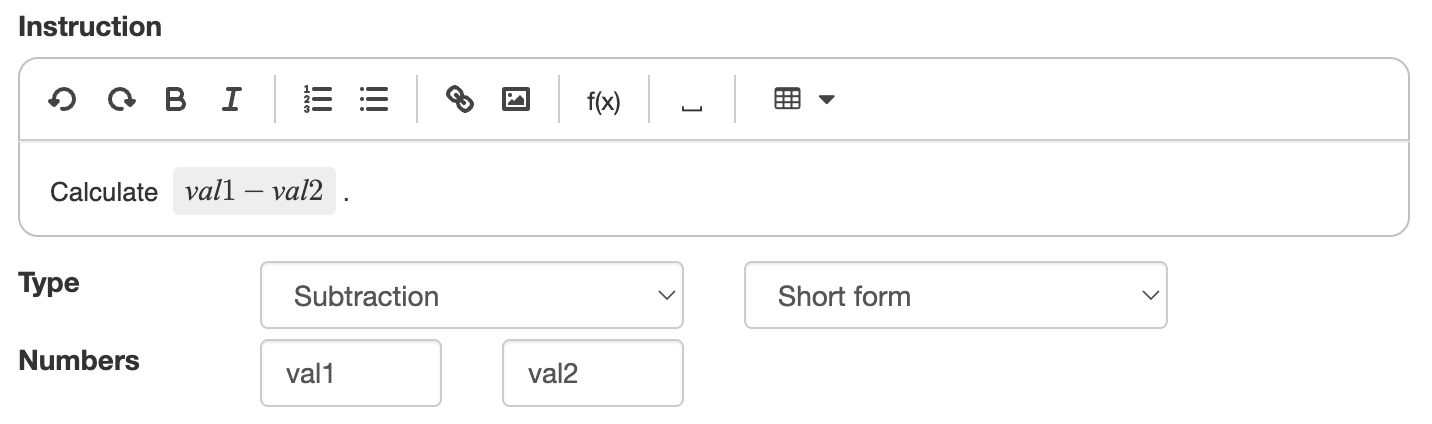# Random multi-digit calculations

## Introduction

You can create random instances of Written Arithmetic questions using the same approach as for Multistep.

• Define exercise parameters in the top bar of the authoring tool
• Use these exercise parameters in the math expressions in the Written Arithmetic question.

## Defining exercise parameters

You create random questions by defining exercise parameters and assigning them random values. You define these exercise parameters in the Randomization panel in the top bar of the exercise. Add the panel with the settings dropdown button on the left if the panel is not already visible.Figure: Accessing the Randomization panel.

The example below defines the values for a random subtraction problem. The first value is an arbitrary integer number between 100 and 999. The second number is always smaller than the first to guarantee a positive solution.Figure: Defining values for a random subtraction problem.

## Using exercise parameters

You can use exercise parameters in any mathematical expression in your exercise. Every time a new question starts, Algebrakit substitutes the exercise parameters with their definitions.Figure: Using exercise parameters in the stem and the question definition.

You can see a live example of this subtraction problem below. There are more examples of division with or without remainder.